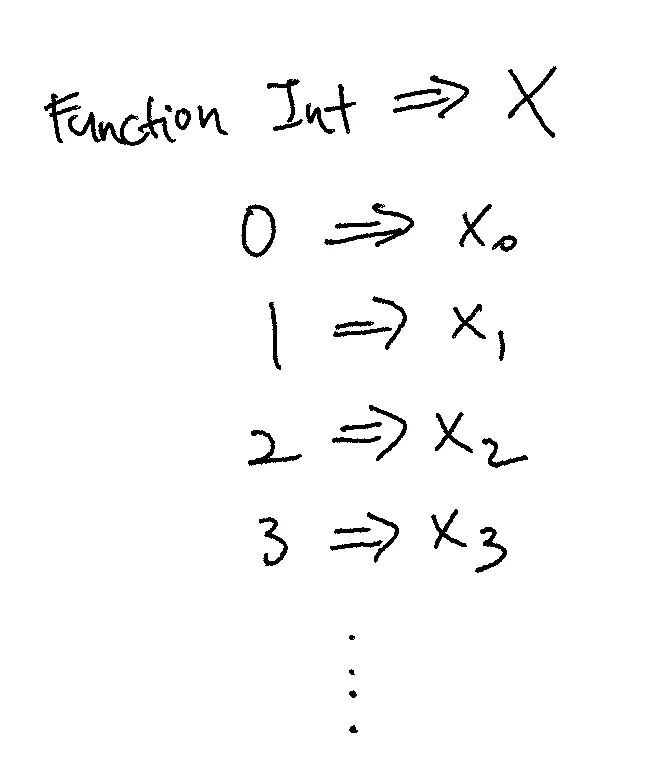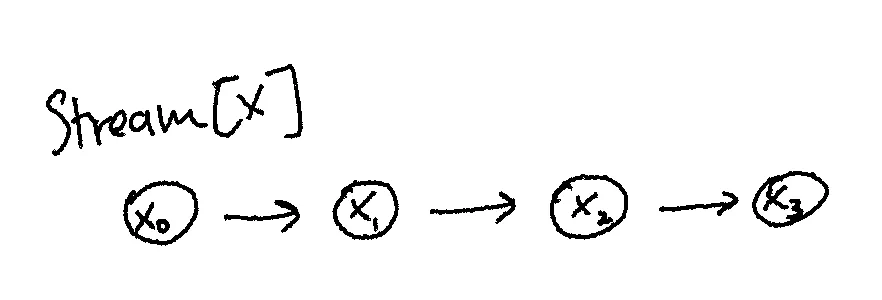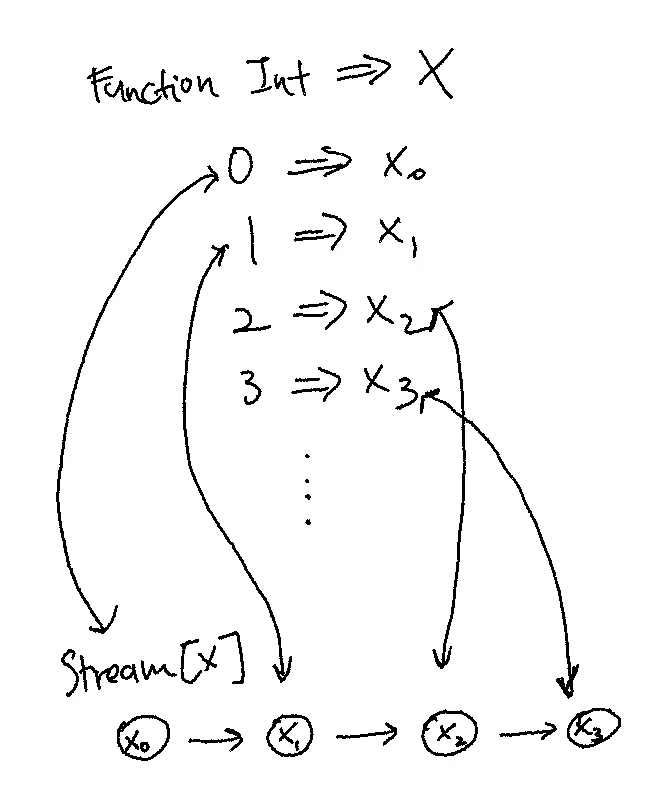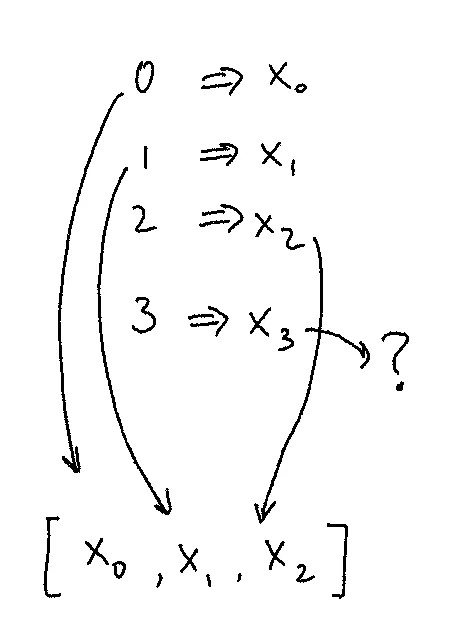# Representable Functors

Posted on:December 23, 2020

It took me a long time to grok representable functors (Chapter 14 of Category Theory for Programmers), so I hope this post will help anyone who’s also struggling. It’ll be a bit silly if I try to motivate everything from scratch, so I’ll assume some familiarity with:

• Morphisms
• Isomorphisms
• Functors

Here we go.

A representable functor is a functor which is isomorphic to the hom-functor.

To unpack that statement, we must first understand how functions and data are really two sides of the same coin.

Imagine a Postgres database storing user data. To keep things simple, let’s say we’re just storing names:

``````CREATE TABLE "user" (
id SERIAL PRIMARY KEY,
name TEXT NOT NULL
);

localhost=# select * from "user";
id | name
----+------
2 | Eve
(2 rows)``````

Given an `id`, we can lookup the user’s name.

Now, it’s not too much of a stretch to imagine converting this Postgres table wholesale into a function that looks like the following:

``````def findUsernameById(id: Int): String = {
else if (id == 2) "Eve"
...
}``````

It sounds contrived, but hopefully you can see how functions are really just compact representations of data. Some people try to use terms like memoization — that once you compute a function with some set of arguments, you can store it, and that is the same thing as looking up a map by key.

Since functors can be thought of containers for data, then for a container to be isomorphic to the hom-functor is to mean that this container can “do” whatever the hom-functor can.

Let’s see an example of a functor which is considered representable: the Stream functor.

``case class Stream[X](h: () => X, t: () => Stream[X])``

Notice that a stream, as compared to a list, cannot be empty, and does not have a definite length.

Let’s also go ahead and define the functor and hom-functor (named here more canonically as `ReaderFunctor`):

``````trait Functor[F[_]] {
def map[A,B](f: A => B): F[A] => F[B]
}

def ReaderFunctor[E](r: E => _) = new Functor[({ type F[X] = E => X })#F] {
def map[A, B](f: A => B): (E => A) => (E => B) = { r => r andThen f }
}``````

Then, we define a Representable trait like so:

``````trait Representable[F[_]] {
type A
def tabulate[B](f: A => B): F[B]
def index[B](f: F[B]): A => B
}``````

We’ll explain more about `tabulate` and `index` in a bit, but notice first of all that the Representable trait contains an abstract type member `A`. This will be our fixed reference point in the category of programming types.

And so, we can implement the Stream functor as a representable functor, using `Int` (assume non-negativity) as the concrete type for A:

``````def representableStream = new Representable[Stream] {
type A = Int
def tabulate[B](f: A => B): Stream[B] = {
Stream[B](() => f(0), () => tabulate(f compose (_ + 1)))
}
def index[B](f: Stream[B]): A => B = f match {
case Stream(x, xs) => {
n => if (n == 0) x() else index(xs())(n - 1)
}
}
}``````

And if you stare at this implementation long enough, what you’ll see is that `tabulate` somehow takes a given function `f`, and “maps” out all results of that function for every input integer 0, 1, 2…, and “stores” that as a stream. Or at least you can think of that way, since it doesn’t actually do the (infinite) computation eagerly, it “stores” it in the tail of the stream.

And `index` is the inverse of that — it takes a stream and unravels it as far as is needed to find the nth item in the stream, which contains the answer to the input n.

Visually, it looks like this:

We have a function, represented here as a lookup table:and we have a `Stream[X]` for some type X:They are really the same thing:Note the bi-directional arrows. We can go between both representations losslessly.

In some sense, what we’re trying to say is, for some function that takes a (non-negative) integer and returns some x in X, a `Stream[X]` is the same thing. They are isomorphic. `tabulate compose index` should be the identity function.

Let’s look at a smaller example. Say we have a `Pair` type, which contains 2 values of some type A:

``case class Pair[A](a: A, b: A)``

If we use `Boolean` as the “key” type, `Pair` is representable, since there are only two possible values for such a function `Boolean => B`. We arbitrarily choose `false` to represent the first item in the `Pair`, and `true` to represent the second item in the `Pair`.

``````def representablePair = new Representable[Pair] {
type A = Boolean
def tabulate[B](f: A => B): Pair[B] = {
Pair[B](f(false), f(true))
}
def index[B](f: Pair[B]): A => B = f match {
case Pair(a, b) => {
n => if (n) f.b else f.a
}
}
}``````

Here, `tabulate` converts a function `Boolean => B` into a `Pair`:

``````scala> representablePair.tabulate((x) => if (x) 1 else 2)
val res0: Pair[Int] = Pair(2,1)``````

And `index` converts a `Pair` into a function `Boolean => B` (here B is a string):

``````scala> val f = representablePair.index(Pair("A", "B"))
val f: Boolean => String = \$anon\$1\$\$Lambda\$1197/1238209644@57cabdc3

scala> f(false)
val res2: String = A

scala> f(true)
val res3: String = B``````

And now circling back to the book, we have:

For F to be representable we require that: There be an object α in C; one natural transformation α from C(α, −) to F; another natural transformation, β, in the opposite direction; and that their composition be the identity natural transformation.

The natural transformation α here is `tabulate`, and β is `index`. The hom-set C(α, −) represents the set of all mappings, and there is a natural transformation from `Reader[A]` to `Stream[A]`.

As an extension, note that the List functor `[]` is not representable, because a list may be empty or too short.